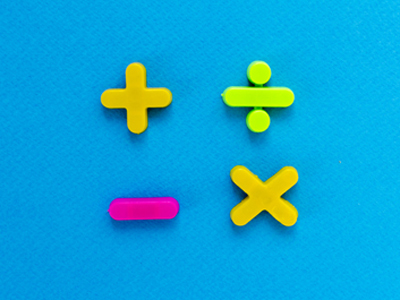# VR - Related Numbers

Related Numbers in the Eleven Plus Verbal Reasoning exam are questions in which children must work out the link between numbers, be it one of addition, subtraction, multiplication or division.

These questions are very rarely found on an Eleven Plus Verbal Reasoning paper, and you can see why! They are mostly a test of maths skills however, they do require a little logic and reasoning, that’s why they are occasionally included.

## How Are Related Numbers Used In The Exam?

Candidates are shown three groups of three numbers, with the middle number in brackets. This middle number is missing from the third set and it’s up to the child to fill in the correct answer.

The numbers in each group are related in the same way and, by applying the same rule to the last group of numbers, the correct answer can be found.

That all sounds very complicated but it’s not as bad as you might think. The best way to show you is with an example:

Example Question One

Look at the relationship between the first and last number in each set of brackets, and use that relationship to find the missing number.

(44  14), (61  23), (79 [?] 26)

To work out the answer, we must find out how 44 and 14 relate to 30. There are only four possible ways: addition, subtraction, multiplication or division. Of these, only one should work. Let’s run through all possible methods:

44 ÷ 14 = 3 remainder 2, not 30. Division is not the relationship.
44 x 14 = 616, not 30, so multiplication is not the relationship.
44 + 14 = 58, not 30, so addition is not the relationship.
44 - 14 = 30. We’ve found the relationship – it’s subtraction.

Next, we apply the same rule to the second set of numbers to see whether or not it holds:

61 - 23 = 38, yes it works. Now we apply it to the last set of numbers to find the answer: 79 - 26 = 53.

Example Question Two

Look at the relationship between the first and last number in each set of brackets, and use that relationship to find the missing number.

(360  15), (629  17), (779 [?] 19)

This question looks a little harder than the first, but it works in exactly the same way. To work out the answer, we must find out how 360 and 15 relate to 24. Let’s try addition, subtraction, multiplication and division in turn. Remember, we can discount any answers which do not equal 24.

360 + 15 = 375
360 - 15 = 345
360 x 15 = 5,400
360 ÷ 15 = 24

So, the relationship is one of division. Next, we apply the same rule to the second set of numbers to see whether or not it holds:

629 ÷ 17 = 37

Yes, it works. Now we know the rule, we can apply it to the third group of numbers to find the missing value:

779 ÷ 19 = 41

This was a particularly hard example and even the most gifted child will have to work out the answer with the help of pencil and paper. However, there will almost certainly be a multiple-choice of answers, which will help speed things along.

There are also a couple of shortcuts we can use to help us. If the middle number is higher than its fellows then we know that the relationship will be either addition or multiplication. If the middle number is lower, then the relationship will be either subtraction or division.

Example Question Three

Look at the relationship between the first and last number in each set of brackets, and use that relationship to find the missing number.

(16  12), (20  17), (11 [?] 27)

Choose the correct answer from the four choices available.
270
297
324
351

In this case, 192 is larger than 16 and 12, whilst 340 is larger than 20 and 17. A quick calculation in your head tells you that 16 + 12 = 28, not 192. Therefore, the relationship must be multiplication.

Now we now the rule, it’s just a case of applying it to the last set of numbers:

11 x 27 = ?

A child who is good at maths will be able to do this in their head: 11 x 27 = (10 x 27) + 27 = 270 + 27 = 297. For the rest of us, we can at least make an estimate: 11 x 27 = something slightly larger than 270.

With no multiple choice, there is no option other than to do the calculation. However, as we have a choice of options, we can discount 270 (too low) 324 and 351 (too high) immediately. That leaves us with the correct answer, 297.

## Sample Tests

As I said earlier, these kind of questions are very rare indeed on a Verbal reasoning Paper. In fact, you’d be more likely to find them on a maths paper. Nevertheless, they can sometimes be included so we have created some tests for you to practise with. Even if your child won’t have to face Related Numbers, you’ll find the quizzes a good way to improve their maths.

There are four quizzes on Related Numbers on the Education Quizzes website. Go through them with your child and show them how to work out the answers. You may find that a calculator helps, but remember that no calculators will be allowed in the exam.

You’ll find all forty questions in the Verbal Reasoning section of Education Quizzes Eleven Plus. Alternatively, you can follow these links:

Related Numbers 1

Related Numbers 2

Related Numbers 3

Related Numbers 4

Good luck!